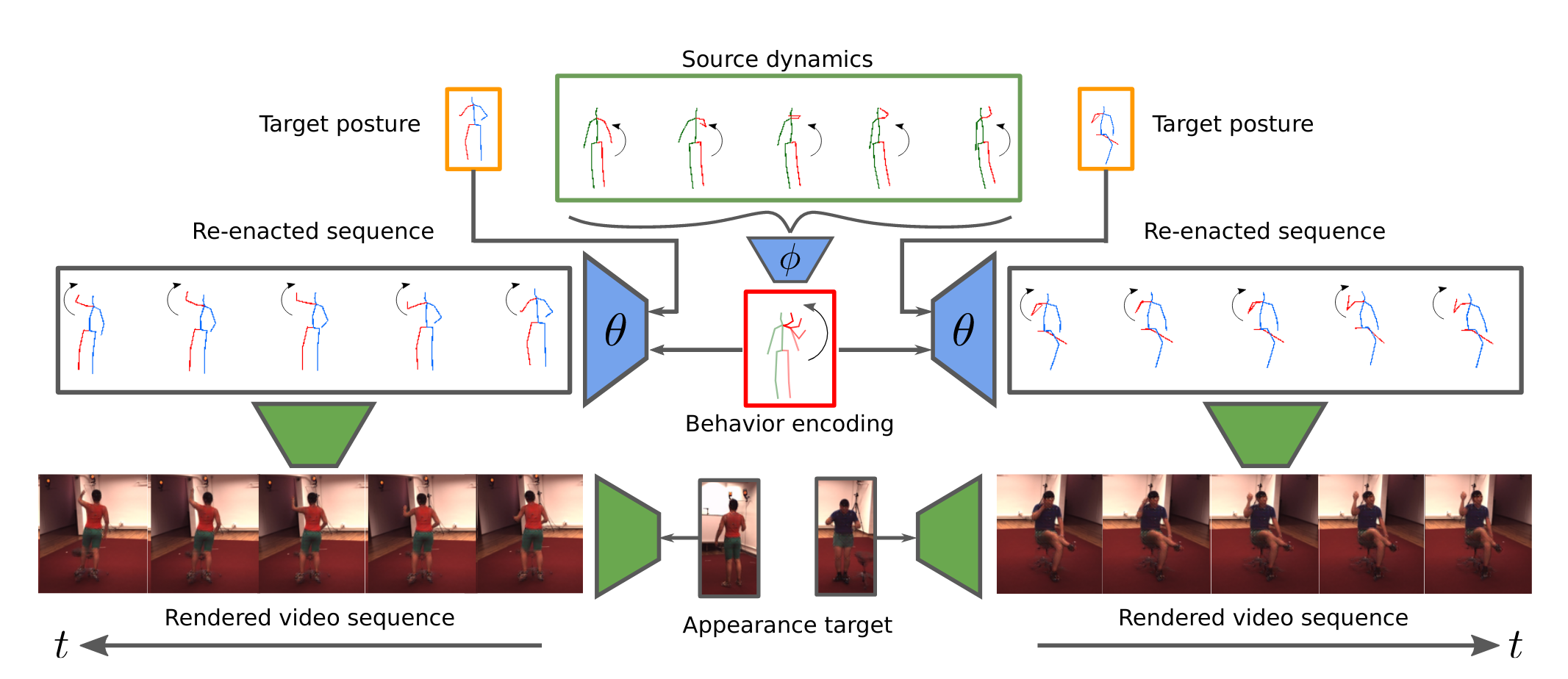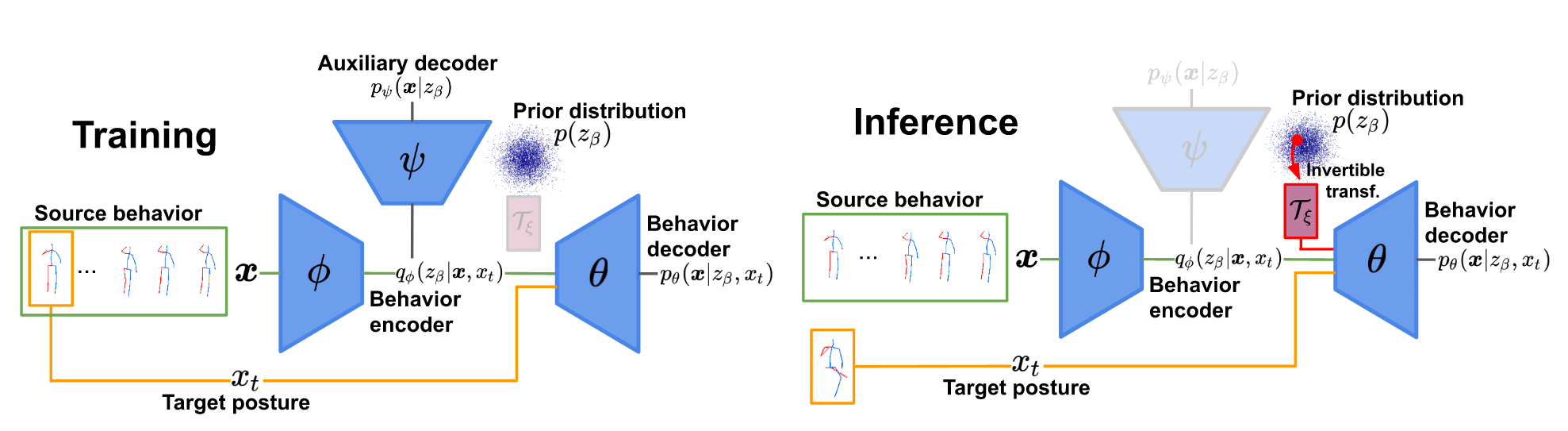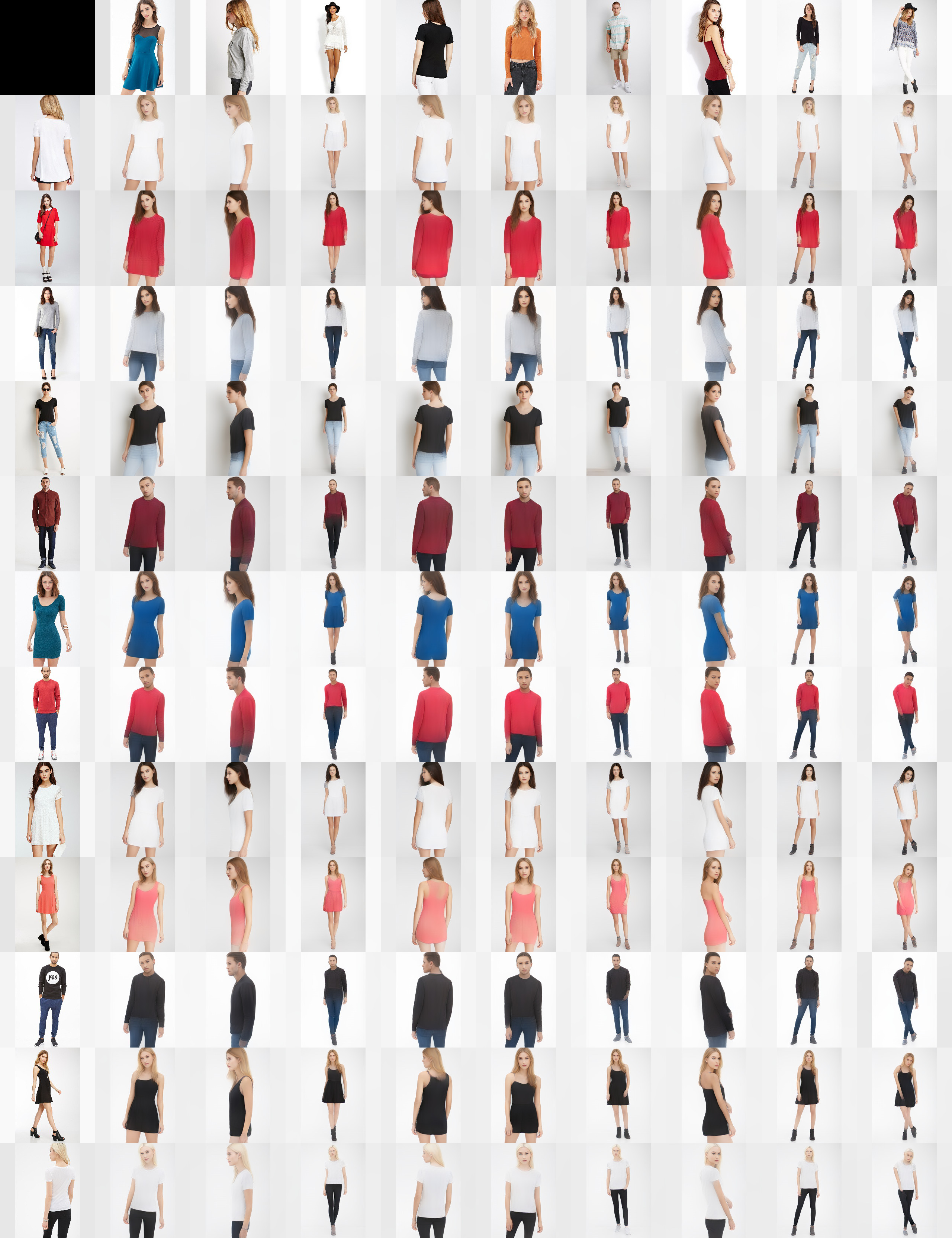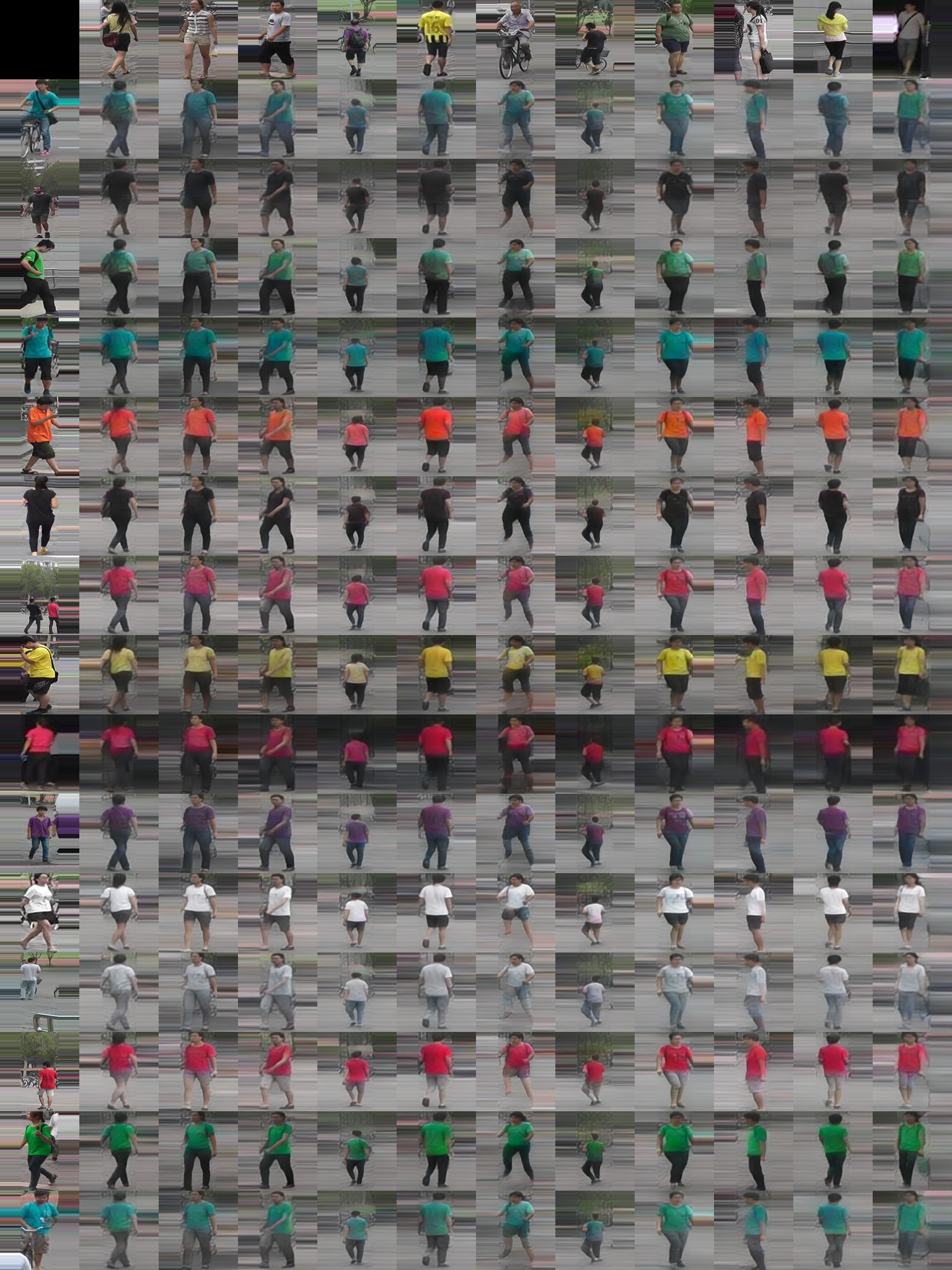TL;DR: Our Approach for Behavior Transfer. Given a source sequence of human dynamics our model infers a behavior encoding which is independent of posture. We can re-enact the behavior by combining it with an unrelated target posture and thus control the synthesis process. The resulting sequence is combined with an appearance to synthesize a video sequence

# Abstract

Generating and representing human behavior are of major importance for various computer vision applications. Commonly, human video synthesis represents behavior as sequences of postures while directly predicting their likely progressions or merely changing the appearance of the depicted persons, thus not being able to exercise control over their actual behavior during the synthesis process. In contrast, controlled behavior synthesis and transfer across individuals requires a deep understanding of body dynamics and calls for a representation of behavior that is independent of appearance and also of specific postures. In this work, we present a model for human behavior synthesis which learns a dedicated representation of human dynamics independent of postures. Using this representation, we are able to change the behavior of a person depicted in an arbitrary posture, or to even directly transfer behavior observed in a given video sequence. To this end, we propose a conditional variational framework which explicitly disentangles posture from behavior. We demonstrate the effectiveness of our approach on this novel task, evaluating capturing, transferring, and sampling fine-grained, diverse behavior, both quantitatively and qualitatively.

## ApproachDuring training we learn an behavior representation $$z_\beta$$ which is independent of posture by combining a special conditional VAE with an auxiliary decoder $$p_\psi$$ measuring the amount of posture present in the behavior sequence. To improve synthesis quality, we relax the latent regularization term in the cVAE-objective and train an invertible transformation $$\mathcal{T}_\xi$$ to close the resulting gap between the prior and posterior distributions in a second stage. In inference mode, we transfer a given source behavior (green) to an arbitrary target posture (yellow) or synthesize novel behavior from the prior distribution which is matched to q by a learned invertible transformation T (red).

## Results

and applications of our model.

## Behavior Transfer

We transfer fine-grained, characteristic body dynamics of an observed behavior $$x_\beta$$ to unrelated, significantly different target postures $$x_t$$. If required, the target posture is first adjusted by a transition phase before re-enacting the inferred behavior.

Visualization of transferred behavior in posture space: Each column shows distinct re-enactements of a given source behavior $$\boldsymbol{x}_\beta$$, visualized in the top-row example, for multiple target postures $$x_t$$.
Visualization of transferred behavior in posture space: Each column shows distinct re-enactements of a given source behavior $$\boldsymbol{x}_\beta$$, visualized in the top-row example, for multiple target postures $$x_t$$.
Visualization of transferred behavior in RGB-space: We generate video sequences by first generating re-enacted posture sequences as explained above. As our proposed disentangling framework can also be applied to posture-appearance transfer (see below for more examples), we can subsequently train a model to disentangle posture from appearance and apply it to generate the individual image frames of a re-enacted posture sequence for a given human appearance. The shown examples visualize this ability based on the synthesized posture sequences depicted above. Note the difference in appearance between the source sequence and the resulting video re-enactments.
Visualization of transferred behavior in RGB-space: We generate video sequences by first generating re-enacted posture sequences as explained above. As our proposed disentangling framework can also be applied to posture-appearance transfer (see below for more examples), we can subsequently train a model to disentangle posture from appearance and apply it to generate the individual image frames of a re-enacted posture sequence for a given human appearance. The shown examples visualize this ability based on the synthesized posture sequences depicted above. Note the difference in appearance between the source sequence and the resulting video re-enactments.

## Behavior Sampling

As we learn a parametric prior behavior distribution $$p(z_\beta)$$, we can use our model to sample novel, unseen behaviors for a given target posture.

To visually evaluate the diversity of behavior sequences sampled by our model we visualize six samples drawn from $$z_\beta$$ for the same target posture in each row.
Nearest neighour visualization in behavior and posture space: We re-enact a source behavior $$\boldsymbol{x}_\beta$$ using a random target posture $$x_t$$. Next, we find its nearest neighbour in the training sequences based on (i) distance between behavior representations $$z_\beta$$ and (ii) average distances between postures sequences (based on alignment w.r.t. the pelvis keypoints). Each column depicts a separate example showing the 'Source Behavior', the 'Nearest Neighbor based on Behavior representation', the 'Behavior Re-enactment of Source Behavior' and the 'Nearest Neighbor based on Posture', i.e. average posture distance. We observe that while gthere exist close training sequences in terms of posture, the nearest neighbors based on $$z_\beta$$ show similar behavior dynamics while being dissimilar in posture.

## Behavior Interpolation

We interpolate between the behavior observed in two sequences $$\boldsymbol{x}_\beta^1$$ and $$\boldsymbol{x}_\beta^2$$. To this end, we first extract their corresponding behavior representations $$z_\beta^1, z_\beta^2$$ and interpolate between them at equidistant steps, i.e. $$(1 - \lambda) \cdot z_\beta^1 + \lambda \cdot z_\beta^2; \; \lambda \in \{0.0,0.2,0.4,0.6,0.8,1.0\}$$. Next, we generate a sequence of interpolated behavior using our decoder $$p_\theta(\boldsymbol{x}|z_\beta,x_t)$$ with $$x_t$$ being the first frame of $$\boldsymbol{x}_\beta^1$$, respectively $$\boldsymbol{x}_\beta^2$$. Note, that for $$\lambda \in \{0,1.0\}$$ we basically reconstruct the source sequences $$\boldsymbol{x}_\beta^1$$, $$\boldsymbol{x}_\beta^2$$.

Interpolated behavior sequences between two sequences $$\boldsymbol{x}_\beta^1$$ and $$\boldsymbol{x}_\beta^2$$.
Video sequences synthesized by our model for the interpolated behavior sequences shown on the left.
Interpolated behavior sequences between two sequences $$\boldsymbol{x}_\beta^1$$ and $$\boldsymbol{x}_\beta^2$$.
Video sequences synthesized by our model for the interpolated behavior sequences shown on the left.
Interpolated behavior sequences between two sequences $$\boldsymbol{x}_\beta^1$$ and $$\boldsymbol{x}_\beta^2$$.
Video sequences synthesized by our model for the interpolated behavior sequences shown on the left.

## Posture-Appearance Transfer

Our approach to disentangling latent representations is not only appicable for factorizing static from temporal information, but also to posture-appearance disentangling. We demonstrate this capability of our model on the DeepFashion and Market1501 datasets.Posture-Appearance transfer on DeepFashion. Our method can also be used to disentangle posture and appearance. A quantitative evaluation can be found Table 1 in the supplementary material.Posture-Appearance transfer on Market1501. Our method can also be used to disentangle posture and appearance. A quantitative evaluation can be found Table 1 in the supplementary material.

## Acknowledgement

The research leading to these results is funded by the German Federal Ministry for Economic Affairs and Energy within the project “KI-Absicherung – Safe AI for automated driving” and by the German Research Foundation (DFG) within project 421703927. This page is based on a design by TEMPLATED.